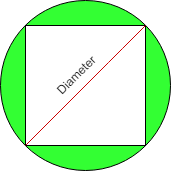Area of square Circumscribed by Circle

• Last Updated : 07 Nov, 2021

Given the radius(r) of circle then find the area of square which is Circumscribed by circle.
Examples:

Input : r = 3
Output :Area of square = 18

Input :r = 6
Output :Area of square = 72

All four sides of a square are of equal length and all four angles are 90 degree. The circle is circumscribed on a given square shown by a shaded region in the below diagram.Properties of Circumscribed circle are as follows:

• The center of the circumcircle is the point where the two diagonals of a square meet.
• Circumscribed circle of a square is made through the four vertices of a square.
• The radius of a circumcircle of a square is equal to the radius of a square.

Formula used to calculate the area of circumscribed square is:
2 * r2
where, r is the radius of the circle in which a square is circumscribed by circle.
How does this formula work?
Assume diagonal of square is d and length of side is a.
We know from the Pythagoras Theorem, the diagonal of a
square is √(2) times the length of a side.
i.e d2 = a2 + a2
d = 2 * a2
d = √(2) * a
Now,
a = d / √2
and We know diagonal of square that are Circumscribed by
Circle is equal to Diameter of circle.
so Area of square = a * a
= d / √(2) * d / √(2)
= d2/ 2
= ( 2 * r )2/ 2 ( We know d = 2 * r )
= 2 * r2

CPP

 // C++ program to find Area of// square Circumscribed by Circle#include using namespace std;  // Function to find area of squareint find_Area(int r){    return (2 * r * r);}  // Driver codeint main() {    // Radius of a circle    int r = 3;          // Call Function to find     // an area of square    cout << " Area of square = "                  << find_Area(r);          return 0;}

Java

 // Java program to find Area of// square Circumscribed by Circleclass GFG {          // Function to find area of square    static int find_Area(int r)    {        return (2 * r * r);    }          // Driver code    public static void main(String[] args)    {        // Radius of a circle        int r = 3;          // Call Function to find        // an area of square        System.out.print(" Area of square = "                             + find_Area(r));    }}  // This code is contributed by Anant Agarwal.

Python3

 # Python program to# find Area of# square Circumscribed# by Circle  # Function to find# area of squaredef find_Area(r):      return (2 * r * r)      # driver code# Radius of a circler = 3      # Call Function to find # an area of squareprint(" Area of square = ", find_Area(r))  # This code is contributed# by Anant Agarwal.

C#

 // C# program to find Area of// square Circumscribed by Circleusing System;  class GFG {          // Function to find area of square    static int find_Area(int r)    {        return (2 * r * r);    }          // Driver code    public static void Main()    {        // Radius of a circle        int r = 3;          // Call Function to find        // an area of square        Console.WriteLine(" Area of square = "                            + find_Area(r));    }}  // This code is contributed by vt_m.



Javascript



Output:

Area of square = 18

Time Complexity: O(1)

My Personal Notes arrow_drop_up